# JP5065113B2 - Equivalence verification method, equivalence verification program, and equivalence verification program generation method - Google Patents

Equivalence verification method, equivalence verification program, and equivalence verification program generation method Download PDF

## Info

Publication number
JP5065113B2
JP5065113B2 JP2008074859A JP2008074859A JP5065113B2 JP 5065113 B2 JP5065113 B2 JP 5065113B2 JP 2008074859 A JP2008074859 A JP 2008074859A JP 2008074859 A JP2008074859 A JP 2008074859A JP 5065113 B2 JP5065113 B2 JP 5065113B2
Authority
JP
Japan
Prior art keywords
model
process
output
fifo
input
Prior art date
Legal status (The legal status is an assumption and is not a legal conclusion. Google has not performed a legal analysis and makes no representation as to the accuracy of the status listed.)
Active
Application number
JP2008074859A
Other languages
Japanese (ja)
Other versions
JP2009230451A (en
Inventor

Original Assignee
ルネサスエレクトロニクス株式会社
Priority date (The priority date is an assumption and is not a legal conclusion. Google has not performed a legal analysis and makes no representation as to the accuracy of the date listed.)
Filing date
Publication date
Application filed by ルネサスエレクトロニクス株式会社 filed Critical ルネサスエレクトロニクス株式会社
Priority to JP2008074859A priority Critical patent/JP5065113B2/en
Publication of JP2009230451A publication Critical patent/JP2009230451A/en
Application granted granted Critical
Publication of JP5065113B2 publication Critical patent/JP5065113B2/en
Application status is Active legal-status Critical
Anticipated expiration legal-status Critical

## Images

•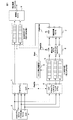•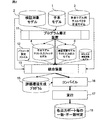•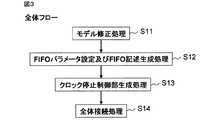•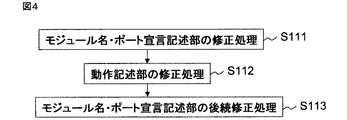••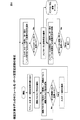•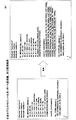•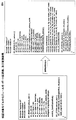•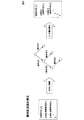••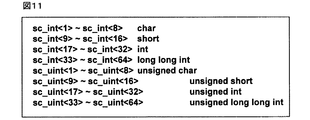•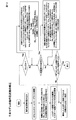•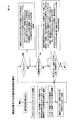•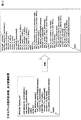•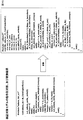•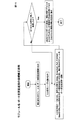•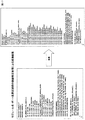•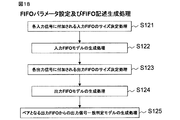•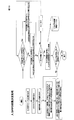•••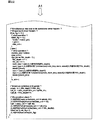•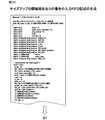•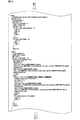••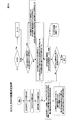•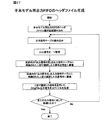•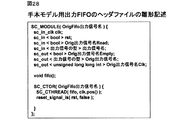••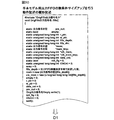•••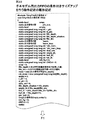••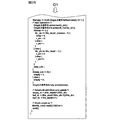•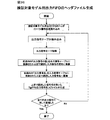•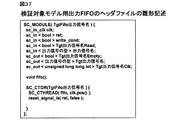•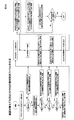•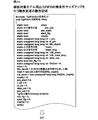•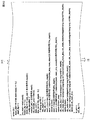•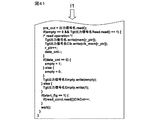•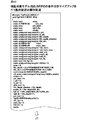•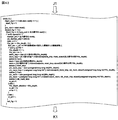•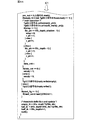•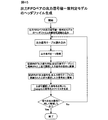•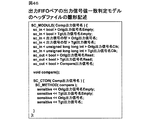•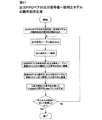•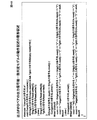•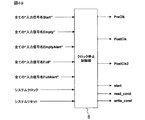••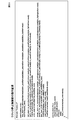•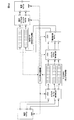## Classifications

• G06F30/3323

## Description

The present invention relates to a technique for generating a verification environment for a new circuit that is developed while maintaining the equivalence of flows (circuit functions and behaviors). For example, the present invention relates to a semiconductor integrated circuit whose functions are expanded while maintaining flow equivalence. The present invention relates to a technology effective when applied to generation of a test environment and a test using the test environment.

Patent Document 1 relates to a technique for determining by simulation the equivalence between an operation level circuit description written in a system level design language and an RTL (Register Transfer Level) circuit description written in HDL (Hardware Description Language). The invention is described. The equivalence determination is performed by individually executing a simulation for each circuit description, determining whether the waveform order of signal value transitions is maintained after acquiring signal waveform information. Therefore, a test bench (a program for generating a test pattern for testing the circuit description) is individually configured for each circuit description, and equivalence determination using the same test bench cannot be realized.

Patent Document 2 focuses on internal processing steps in behavioral synthesis and is intended to check whether changes and operations in each internal processing are performed correctly, so that equivalence determination before and after synthesis is performed. The invention is described. This proves that the description change is correctly performed in each deformation process of the input description performed by the behavioral synthesis tool, and directly accesses the intermediate expression generated by each deformation process. EFSM (Extended Finite State Machine) is extracted from the intermediate representation before and after the transformation process, the next state function and output function of each EFSM are obtained by Symbolic Simulation, and it is determined whether the obtained next state function and output function are equal. Equivalence verification is performed by verifying. That is, the technique described in Patent Document 2 is not an equivalence verification method by simulation using a test bench.

Patent Document 3 aims to enable equivalence verification by comparing the input description to behavioral synthesis and the simulation result waveform of the output result RTL, so that equivalence judgment before and after synthesis is performed. Described invention is described. In this invention, the behavioral synthesis input description and the output result RTL are individually simulated, and the correspondence of the transaction in each output waveform is identified using the allocation cycle designation information for the input description or the cycle information inserted by behavioral synthesis. In addition, the equivalence verification of the input description and the output description is performed by comparing the output waveform in units of transactions. Therefore, a test bench description is required for each of the input description and the output result RTL.

JP 2005-316595 A Japanese Patent Laid-Open No. 2003-141202 JP 2004-145712 A

The present inventor has studied diverting a test bench for realizing a test environment for a basic circuit model to verify equivalence of a newly developed circuit with respect to the basic circuit model. None of the above Patent Documents 1 to 3 is suitable for diverting a test bench in that sense. As described above, in the technique of Patent Document 1, equivalence determination using the same test bench for each circuit description of the behavior level circuit description described in the system level design language and the RTL circuit description described in HDL. Cannot be realized. The technique of Patent Document 2 is not an equivalence verification method by simulation using a test bench in the first place. In the technique of Patent Document 3, a test bench description is required for each of the input description and the output result RTL.

The present inventor cannot easily divert the test bench before and after the synthesis, because the scheduling in the behavioral synthesis makes the input and output access timing cycle of the synthesis input description and the input and output access of the synthesis result. We focused on the fact that it was caused by the difference in timing cycle. Since the input and output access timing cycles are different before and after the synthesis, the test bench used in the synthesis input description cannot be used as it is. It is expected that the number of man-hours will increase significantly when the result of synthesis is analyzed and correction is performed manually so that connection is possible.

An object of the present invention is to provide a verification method in which a test bench for a basic circuit model is used for verification of equivalence of a newly developed circuit with respect to the basic circuit model.

Another object of the present invention is to generate a verification environment for enabling verification of equivalence to an RTL description model or a simulation model obtained using a behavioral synthesis tool by using an existing test bench. Is to provide.

Still another object of the present invention is to generate a verification environment for enabling the examination of equivalence to an RTL description model or a simulation model obtained by using a behavioral synthesis tool by using an existing test bench. It is to provide a method.

The above and other objects and novel features of the present invention will be apparent from the description of this specification and the accompanying drawings.

The following is a brief description of an outline of typical inventions disclosed in the present application.

That is, in order to verify the equivalence of the model to be verified using a model model in which a circuit is described in a predetermined language and a test vector generation model for the model model, the model model has cycle accuracy. Input / output of signals from / to the test model generation model for the model model, and input the output from the test vector generation model for the model model for each signal of the model model at the same timing as the model model A process of writing to the FIFO group, a process of reading data from the input FIFO group at the same operation timing as the verification target model and outputting the data to the verification target model, and outputting the example model and the output of the verification target model; Is written to the output FIFO pair group for each corresponding signal name, and a match determination is performed for the pair output for each written signal name.

Thereby, an input and output access timing cycle between the model model and a test vector generation model as a test bench thereof, and an input and output access timing cycle between the verification target model and the test vector generation model, Are absorbed by the input FIFO group and the output FIFO pair group. Therefore, the model model test bench can be used for the equivalence evaluation of the verification target model.

The effects obtained by the representative ones of the inventions disclosed in the present application will be briefly described as follows.

That is, the test bench for the basic circuit model can be used for verifying the equivalence of a newly developed circuit with respect to the basic circuit model.

1. First, an outline of a typical embodiment of the invention disclosed in the present application will be described. Reference numerals in the drawings referred to in parentheses in the outline description of the representative embodiments merely exemplify what are included in the concept of the components to which the reference numerals are attached.

 An equivalence verification method according to a specific form of the present invention is a verification target using a model model in which a circuit is described in a predetermined language and a test vector generation model for the model model. This is a method for verifying equivalence of models, and includes a first process to a fifth process as processes executed by a computer device. The first process is a process in which the model model inputs / outputs signals with the model model test vector generation model with cycle accuracy. The second process is a process for performing control to write the output from the test model generation model for the model model into the input FIFO group for each signal of the model model at the same timing as the model model. The third process is a process of reading data from the input FIFO group at the same operation timing as the verification target model and outputting the data to the verification target model. The fourth process is a process of writing the output of the model model and the output of the verification target model into the output FIFO pair group for each corresponding signal name. The fifth process is a process of performing coincidence judgment on the pair output for each signal name of the output FIFO pair group.

Thereby, an input and output access timing cycle between the model model and a test vector generation model as a test bench thereof, and an input and output access timing cycle between the verification target model and the test vector generation model, This difference is absorbed by the second to fourth processes using the input FIFO group and the output FIFO pair group. Therefore, the model model test bench can be used for the equivalence evaluation of the verification target model.

 In the equivalence checking method according to item 1, for example, in the first process and the second process, all the input FIFOs of the input FIFO group are set to a first input FIFO threshold (D (port) / 2, D (port ) X (N-1) / N, D (port) / N, a value selected from an arbitrarily specified positive number) or when one of the input FIFOs is held. Execution is stopped when it becomes full and executed in other states. When all the input FIFOs hold more data than the number specified by the first input FIFO threshold, the execution of the first and second processes is stopped, so that each input FIFO is randomly and The state of frequent fullness can be alleviated, which can contribute to improvement in verification processing efficiency.

 In the equivalence checking method according to item 1, for example, the third process and the fourth process are executed after all the input FIFOs in the input FIFO group hold a predetermined number of data or more. Be started. This is because the difference in input and output access timing cycles between the model model and the model to be verified is absorbed in advance before the processing is started, so that the efficiency of the verification processing is improved.

 In the equivalence checking method according to item 3, in the third process and the fourth process started to be executed, all the input FIFOs of the input FIFO group are set to a second input FIFO threshold (D (port) / 2, D (port) x (N-1) / N, D (port) / N, a value selected from an arbitrarily specified positive number) or less than the specified number of data, or the input FIFO When even one of them is empty, the execution is stopped and executed in other states. It is possible to alleviate the state in which individual input FIFOs are randomly and frequently empty, which can contribute to improvement in verification processing efficiency.

 The equivalence checking method according to  further includes a size-up process for increasing the number of FIFO stages each time the number of data held in the input FIFO reaches a predetermined number as a process executed by the computer apparatus. Since the number of input FIFO stages can be dynamically increased in response to signals that are frequently input and output, the possibility of a specific FIFO becoming full can be reduced, which contributes to improved verification processing efficiency. be able to.

 In the equivalence verification method of item 5, when the number of data held in the input FIFO reaches a predetermined number, for example, the difference between the read pointer and the write pointer value of the input FIFO is the third input FIFO threshold (D ( port) / 2, D (port) × (N−1) / N, D (port) / N, a value selected from an arbitrarily specified positive number). Whether or not the size can be increased can be controlled in accordance with the difference in operation speed between writing and reading to the input FIFO.

 In the equivalence checking method according to item 6, for example, the third input FIFO threshold is a number that is increased according to a variable (N) that is increased for each size-up process. It becomes easy to appropriately control whether or not the next size increase once the size has been increased.

 The equivalence checking method according to  further includes a size-up process for increasing the number of FIFO stages each time the number of data held in the output FIFO reaches a predetermined number as a process executed by the computer apparatus. Since the number of output FIFO stages can be increased dynamically in response to signals that are frequently input and output, the possibility of a specific output FIFO becoming full can be reduced, and verification processing efficiency can be improved. Can contribute.

 In the equivalence checking method according to item 8, for example, when the number of data held in the output FIFO reaches a predetermined number, the difference between the read pointer and the write pointer value of the output FIFO is the output FIFO threshold (D (port) / 2, D (port) × (N-1) / N, D (port) / N, a value selected from an arbitrary positive number). Whether or not the size can be increased can be controlled in accordance with the difference in the operation speed between writing to and reading from the output FIFO.

 In the equivalence checking method according to item 9, the output FIFO threshold is a number that is increased according to a variable (N) that is increased for each size-up process, for example. It becomes easy to appropriately control whether or not the next size increase once the size has been increased.

 An equivalence verification program according to another specific form of the present invention is verified using a model model in which a circuit is described in a predetermined language and a test vector generation model for the model model. This is a program for causing a computer device to execute first to fifth processes for verifying equivalence of a target model. The first process is a process in which the model model inputs / outputs signals to / from the model model test vector generation model with cycle accuracy. The second process is a process of performing control to write the output from the test model generation model for the model model into the input FIFO group for each signal of the model model at the same timing as the model model. The third process is a process of reading data from the input FIFO group at the same operation timing as the verification target model and outputting the data to the verification target model. The fourth process is a process of writing the output of the model model and the output of the verification target model into the output FIFO pair group for each corresponding signal name. The fifth process is a process of performing a match judgment on the pair output for each signal name of the output FIFO pair group.

By executing this equivalence verification program on a computer device, it becomes possible to easily evaluate the equivalence of the verification target model by diverting the model model test bench.

 In the equivalence checking program according to item 11, for example, in the first process and the second process, all the input FIFOs in the input FIFO group hold more than the number of data defined by the first input FIFO threshold. When this happens, or when one of the input FIFOs becomes full, the execution is stopped and executed in other states.

 In the equivalence checking program according to item 11, for example, the third process and the fourth process wait for all the input FIFOs in the input FIFO group to hold a predetermined number or more of data. Execution starts.

 In the equivalence verification program according to item 13, for example, the third process and the fourth process started to be executed are equal to or less than the number specified by the second input FIFO threshold for all the input FIFOs in the input FIFO group. When the data is held or when one of the input FIFOs is empty, the execution is stopped and executed in other states.

 The equivalence checking program according to item 11 further includes a size-up process for increasing the number of FIFO stages each time the number of data held in the input FIFO reaches a predetermined number as a process executed by the computer device.

 In the equivalence checking program according to item 15, for example, when the number of data held in the input FIFO reaches a predetermined number, the difference between the read pointer and the write pointer value of the input FIFO is equal to or greater than a third input FIFO threshold. Is to become.

 In the equivalence checking program according to item 16, the third input FIFO threshold is a number that is increased according to a variable that is increased for each size-up process, for example.

 The equivalence checking program according to item 11 further includes a size-up process for increasing the number of FIFO stages each time the number of data held in the output FIFO reaches a predetermined number, for example, as a process executed by the computer apparatus.

 In the equivalence checking program according to item 18, when the number of data held in the output FIFO reaches a predetermined number, for example, the difference between the read pointer and the write pointer value of the output FIFO becomes equal to or greater than the output FIFO threshold. It is.

 In the equivalence checking program according to item 19, the output FIFO threshold is a number that is increased according to a variable that is increased for each size-up process, for example.

 A method for generating an equivalence checking program according to still another specific embodiment of the present invention includes a model model in which a circuit is described in a predetermined language, a test vector generation model for the model model, Is a method for generating an equivalence verification program for verifying equivalence of a model to be verified, and includes first to sixth processes as processes executed by each computer device. The first process is a process of reading the sample model, the test vector generation model, and the parameter setting file into a computer device. The second process is a process of generating a model model test input fetch model for performing an operation of writing data input from the model model test vector generation model to the input FIFO group with cycle accuracy. The third process is a process of generating a verification target model test input / output model for performing an operation of reading data from the input FIFO group and outputting the data to the verification target model according to the operation timing of the verification target model. The fourth process is a process of generating a model of the input FIFO group based on the model model test input fetch model and the verification target model test input output model. The fifth process is a process of generating an output FIFO pair group that receives and holds the output of the model model and the output of the verification target model for each corresponding signal name. The sixth process is a process for generating a coincidence determination model for performing the coincidence determination of the output pair for each signal name of the output FIFO pair group.

By using this method, it becomes possible to easily obtain an equivalence verification program for evaluating the equivalence of the verification target model by diverting the test bench of the model model.

2. Details of Embodiments Embodiments will be further described in detail. DESCRIPTION OF EMBODIMENTS Hereinafter, embodiments for carrying out the present invention will be described in detail with reference to the drawings. Note that components having the same function are denoted by the same reference symbols throughout the drawings for describing the embodiments for carrying out the invention, and the repetitive description thereof will be omitted.

<< Outline of test environment using existing test bench >>
FIG. 1 illustrates the configuration of a virtual test environment generated by the test environment generation method of the present invention. The test environment shown in the figure enables verification of the function of a model 2 to be verified that can be matched between ports with respect to the model 1 using the test vector generation model 3 for the model. is there. The model model 1 and the verification target model 2 have port names that correspond one-to-one with each other and can obtain an output signal sequence equivalent to the input signal sequence when time or timing is ignored. (Input / output equivalence) is assumed. For example, the verification target model 2 has a relationship in which internal processing is pipelined with respect to the model model 1 or execution cycles are added to internal operations. The test vector generation model 3 for the model model is a test bench for verifying the function of the model model, and the test environment of FIG. 1 verifies the verification target model by diverting the model model test bench. Make it possible.

The test bench is configured as a program that can be executed by a computer device such as an EWS (Engineering Work Station). In FIG. 1, a model model 1, a verification target model 2, a test vector generation model 3 for the model model, a model model test input fetch model 4, an input FIFO group 5, a verification target model test input output model 6, and an output FIFO Each of the pair group 7, the clock stop control unit 8, and the coincidence determination model 9 is positioned as a functionally divided program module. Each is executed by a computer device.

The model model 1 performs signal input / output with the model vector test vector generation model 3 with cycle accuracy. The model model test input fetch model 4 fetches the output from the model generation test model generation model 3 for the model model at the same timing as the model model 1, and the FIFO of the corresponding signal name (port name) of the input FIFO group 5 (Push) control is performed. The verification target model test input output model 6 reads (pops) and fetches input data from the input FIFO group 5 at the same timing as the verification target model 2, and outputs the fetched data to the verification target model 3. The output FIFO pair group 7 holds the output of the model model 1 and the output of the verification target model 2 for each signal name. The FIFO of the output FIFO pair group 7 is pushed when the input value changes. The clock stop control unit 8 controls each component of the test environment so that the input FIFO group 5 does not become full or empty. When both of the output FIFO pairs of the output FIFO pair group 7 have poppable data at the head, the coincidence determination model 9 fetches data from the FIFO pair and performs data coincidence comparison. This makes it possible to verify the function of the verification target model 2 having input / output equivalence with respect to the model model 1 by using the test bench of the model model. If cycle information is also stored in the output FIFO pair group 7, it is possible to determine which part of the simulation result waveform should be compared when a mismatch in the output data is detected by the coincidence determination model. Verification in consideration is also possible. Also, by checking the cycle information, it is possible to check whether the expected cycle deviation has occurred. Hereinafter, the test environment generation method and the like will be described in detail.

<< Outline of test environment generation process >>
FIG. 2 shows an outline of the test environment generation process. The model model 1, the verification target model 2, and the model model test vector generation model 3, which are two models with different levels of abstraction that can be handled between ports, are input to the program correction device 11. For example, it is assumed that the model model 1 is described in a system level language such as a system C (SystemC), and the verification target model 2 is described in a system level language or HDL (hardware description language). In particular, when the model model 1 or the verification target model 2 is a model described in HDL, the model model 1 or the verification target model 2 described in a system description language whose operation matches with the HDL description at the cycle level. Is also an input. Here, the HDL description of the verification target model 2 is an RTL obtained as a result of specifying an assignment cycle on the program path for the model model 1 or synthesizing with a behavioral synthesis tool by specifying pipelining. (Register transfer level) description may be used. Further, in many cases, commercially available behavioral synthesis tools have a function of generating a verification target model described in a system description language whose behavior matches that of the RTL at the cycle level. It is assumed that it was generated using

The program correction device 11 generates a model model test input fetch model 4 for writing the data to the input FIFO group 5 immediately after fetching the input data from the model model 1 described in the system level language. Alternatively, the verification target model test input / output model 6 for generating the input data to the verification target model 2 by generating the input data to the verification target model 2 described in HDL from the input FIFO group is generated.

The integration device 13 includes the sample model 1, the verification target model 2, the sample model test vector generation model 3, the sample model test input fetch model 4, the verification target model test input output model 6, and the parameter setting file 12. Based on these, the input FIFO group 5, the output FIFO pair group 7, and the coincidence determination model 9 are generated and all the generated models are integrated to generate the evaluation environment generation program 15 described in FIG. . The evaluation environment generation program 15 is compiled and converted into an execution code (16). The computer apparatus executes the evaluation environment generation program 15 via the execution code (17), and can obtain a match or mismatch with the output of the model model 1 with cycle accuracy for each output port of the evaluation target model 2 (18). ). When the model model 1 or the verification target model 2 is HDL description, it is preferable to use a co-simulation function of a commercially available simulator. Therefore, the integration in the integration device 13 also means that some models are integrated so that they can be evaluated using the co-simulation function.

By executing the evaluation environment generation program 15, the input model sequence fetched from the test model generation model 3 for the model model by the model model 1 is transferred to the input FIFO group 5 by the model model test input fetch model 4. The verification target model test input / output model 6 performs data fetching at the timing when the verification target model 2 fetches input data from the input FIFO group 5 and inputs data to the verification target model 2. Each output port of the model model 1 and the model 2 to be verified is provided with an output FIFO pair 7, and the output FIFO pair 7 has a value only when the output value from the corresponding output port of the model model changes. Store. While data is stored at the head of both FIFOs of the output FIFO pair 7, the coincidence determination model 9 performs a value comparison, and the output of the model model 1 and the output of the verification target model 2 match or do not match. That is, equivalence determination is performed. The clock stop control unit 8 acquires full information and empty information from the input FIFO group 5 to control the clock of each model so that a full or empty state in the input FIFOI group 5 does not occur, that is, operation stop control and Performs operation resumption control. The parameter setting file 12 is used to specify the FIFO initial stage number of the input FIFO group 5 and the output FIFO pair 7, the manner of changing the FIFO stage number, and the like. If there is no such designation, it is assumed that the default value is set by the integration device 13.

The port may be a pin accuracy or a TL (Transaction Level) port as long as the correspondence can be made between the model model and the verification target model.

<Flow equivalence>
The flow equivalent of two circuits and functional blocks (processes) means that when time is ignored, when equivalent input data series is input to each input port of both processes, both times are ignored. This means that the data series obtained from each output port of the process corresponds between the processes. For example, if the input port group and output port group in each process and the order of input / output and execution order are the same in the two processes ignoring the time, the input data fetched state and output respectively. When a state transition graph is constructed by linking the states where the data values have changed based on the execution order, these state transition graphs are mutually time abstracted bisimilar. Equivalent). In the overall configuration of the test environment disclosed in the present application, full empty control is performed in the FIFO such as the input FIFO group 5, but this operation only prevents the output value of each process from changing at the same timing. Since the equivalence determination is performed based on the change in the value, the equivalence determination is not affected. Details on the concept of equivalence can be found in, for example, the literature “Paul Le Guernic, Jean-Pierre Talpin, and Jean-Christophe Le Lann,“ POLYCHRONY FOR SYSTEM DESIGN, ”Journal of Circuits, Systems, and Computers, 12 (3). , pp.261-304, 2003 ”.

《Description of model model》
When the input description of the model model 1 is, for example, an operation description by the system C described with cycle accuracy, the model model test vector generation model 3 is a test bench for the operation description by the system C. It is assumed that both the model model 1 and the model model test vector generation model 3 are connected and can be simulated.

<Description of model to be verified>
For example, when the model model 1 is created as a behavior description by the system C with cycle accuracy, the verification target model 2 to be compared in the operation matching verification is a pipeline of the internal loop, a pipeline of the entire process, a program A behavioral description obtained by arbitrarily applying a cycle assignment to a program path from a certain point to a certain point, or an RTL obtained by arbitrarily performing the above three items by behavioral synthesis. The behavioral description of the verification target model 2 can be acquired as a system C description output as a simulation model of an RTL as a synthesis result by using a commercially available behavioral synthesis tool or the like.

<Parameter setting file>
The parameter setting file 12 defines parameters for the FIFO of the input FIFO group 5 (also simply referred to as input FIFO) and parameters for the FIFO of the output FIFO pair 7 (also simply referred to as output FIFO).

The parameter of the input FIFO group 5 is first the number of FIFO stages. This is a parameter for setting the number of FIFO stages arranged corresponding to each input port. This parameter is used as a multiplier in the FIFO stage number calculation in the input FIFO stage number determination process described separately. If not specified, for example, 2 is adopted as the default value. It may be arbitrarily specified. Secondly, the setting is related to the contents of notification to the clock stop control unit 8. The notification contents to the clock stop control unit 8 include a certain amount to stop the operation clock on the pop side of the FIFO until the amount of data stored in the FIFO (the number of FIFO data storage stages) reaches a certain amount after the reset is released. Notification that the amount of accumulated data in the FIFO has exceeded a certain amount, and notification that the amount of accumulated data in the FIFO has become less than a certain amount. A setting parameter is set to N, and using N, a parameter necessary for the notification is determined by input FIFO threshold value setting means to be described later. When N is not designated, for example, 4 is adopted as a default value. Third, there is a setting related to FIFO size increase. This is a parameter relating to the dynamic increase in the number of FIFO stages arranged corresponding to each input port. The mode that can be set by the parameter is that when the value of the write pointer to the FIFO reaches, for example, the FIFO size or a value in the vicinity thereof, it is unconditionally increased to double the number of stages or “write pointer−read pointer ≧ input described later” In the case of “FIFO threshold”, the number of stages is doubled. In particular, when the input FIFO threshold value described later is not specified, the former is selected.

The parameter of the output FIFO pair 7 is firstly the number of FIFO stages. This is a parameter for setting the number of FIFO stages arranged corresponding to each output port. Based on this parameter value, the number of individual FIFO stages is determined in an output FIFO stage number determination process described separately. If not specified, for example, 8 stages are adopted as default values. It may be arbitrarily specified. Second, there is a setting related to FIFO size increase. This is a setting parameter for increasing the number of FIFO stages arranged corresponding to each input port. When the value of the write pointer to the FIFO reaches the FIFO size or a value in the vicinity thereof, it is unconditionally increased to double the number of stages, or when “write pointer−read pointer ≧ input FIFO threshold value described later” is doubled. The number of stages is either increased. In particular, when the input FIFO threshold value described later is not specified, the former is selected.

<< Overall generation flow of evaluation environment generation program >>
FIG. 3 illustrates the overall generation flow of the evaluation environment generation program. The generation flow includes model correction processing (S11), FIFO parameter setting and FIFO description generation processing (S12), clock stop control unit generation processing (S13), and overall connection processing (S14).

The model modification process (S11) generates a model model test input fetch model 4 for writing the data to the input FIFO group immediately after fetching the input data based on the model model 1 described in the system description language. This is processing for generating a verification target model test input output model 6 for outputting input data obtained by fetching input data from the input FIFO group 5 based on the verification target model 2 described in a description language.

The FIFO parameter setting and FIFO description generation process (S12) sets the number of FIFO stages of each FIFO of the input FIFO group 5 and the output FIFO pair 7, and the FIFO remaining amount necessary for performing signal notification to the clock stop control unit 8. This is a process of generating a description of the input FIFO group 5 and the output FIFO pair 7 in which the number of stages increase mode is set.

In response to the signal notification from the input FIFO group 5, the clock stop control unit generation process (S13) is a pre-stage comprising the model model 1, the model model test vector generation model 3, and the model model test input fetch model 4. This is a process of generating a description of a module for controlling the clock supply stop and the clock supply restart to the module group, and the subsequent module group including the verification target model 2 and the verification target model test input output model 6.

The whole connection process (S14) includes the model obtained by the model correction process, the input FIFO group 5, the output FIFO pair 7, the clock stop control unit 8, the model model 1, the verification target model 2, and the coincidence determination model 9. It is a process to connect.

《Model correction processing》
Details of the model correction processing (S11) will be described. As illustrated in FIG. 4, the model correction process (S11) is a module name / port declaration description part correction process (S111), an operation description part correction process (S112), and a module name / port declaration description part. It consists of a correction process (S113).

First, the module name / port declaration description part correction process (S111) will be described. FIG. 5 illustrates details of the modification process (S111) of the module name / port declaration description part for the model model 1. This process is a process for correcting the header description. That is, in this modification process, the module name / port declaration description part of the model model description is input, the module name, input port declaration and output port declaration are identified, the module name is changed, and each input port variable is changed. First, a function prototype declaration of the input FIFO corresponding to each input port is defined, and the function prototype declaration of the newly defined input FIFO is added to the port declaration description part. Change the identified output port declaration to a signal variable declaration. The identified input signal name and output signal name are stored in the input signal name table and the output signal name table, respectively. The signal type is also registered in the output signal name table with reference to the correspondence table in FIG. The file name of the obtained module name / port declaration description part is set as “_original file name” and stored in the input signal table.

It is assumed that the prototype declaration of the input FIFO function is given by “void fifo_input signal name (char rst, char rw_flg, input signal type wdata, input signal type * rdata);”. Therefore, when a prototype declaration is added, it is assumed that information on the input signal name and input signal type is obtained from the information on the identified input port and is replaced as appropriate. The meaning of the input FIFO function argument, char rst, char rw_flg, input signal type wdata, input signal type * rdata is as follows:
char rst; // 0 for initialization, 1 for normal operation,
char rw_flg; // 1 reads FIFO, 0 writes FIFO,
Input signal type wdata; // If rw_flg is set to 1, wdata is pushed to the FIFO,
Input signal type * rdata; // If rw_flg is set to 0, data is popped from the FIFO and reflected in rdata.

FIG. 6 illustrates details of the modification process (S111) of the module name / port declaration description part for the model 2 to be verified. In this modification process, the module name / port declaration description part of the model description to be verified is input, the input port declaration and function prototype declaration are identified, a new function is defined based on the function prototype declaration, and each input port variable is defined. Originally define a new output port variable, and add the newly defined output port variable declaration and function prototype declaration to the module name port declaration description part ("_original file name") of the modified model model . If there are member local variable declarations, identify them, store them in the member local variable table, and add a variable declaration with the variable name of each local variable as “_local variable name”.

FIG. 7 illustrates the result of converting the module name / port description part of the model 1 according to the procedure of FIG. The underlined part is the description part added by conversion.

FIG. 8 illustrates the module name / port description part of the verification target model 2 and the result of conversion according to the procedure of FIG. The underlined portion in FIG. 8 is a description portion embedded in the conversion result in FIG.

Next, the behavior description correction process (S112) will be described. FIG. 9 conceptually shows a common process for both the model model and the function verification model for correcting the behavioral description. First, each control flow graph (CFG) is expanded, and if (conditional expression) -else if (conditional expression) branch structure is changed to if (conditional expression) -else {if (conditional expression)} branch structure as shown in the figure. Perform conversion on the code.

FIG. 10 shows a flowchart of processing for converting from the model model to the behavioral description of the model model test input fetch model, including the conversion processing shown in FIG. Reads the input signal variable name and type information from the input signal name table, and operates the local variable declaration description of the same type as "tmp_input signal name" or the type defined based on the next item for each input signal. Insert in the description section. In particular, when the variable type of the input signal variable is the SystemC data type of sc_uint <T> or sc_int <T>, the type of the local variable in the function declared as “tmp_input variable name” using the correspondence table of FIG. Shall be defined. This type determination operation is performed similarly in the output FIFO.

FIG. 12 shows a specific example of the modification process of the behavior description part for the model model 1. The behavioral description of the model model is read, and the initialization description of the FIFO function generated for each input port is embedded in the initialization description part of the behavioral description. In the internal behavioral description part, the value read from the input port is By substituting for the variable “tmp_input signal name” and passing the variable to the argument of the FIFO function, the description for embedding the input signal value to the FIFO is embedded. FIG. 14 illustrates the behavior description part of the model model and the conversion result by the processing of FIG.

FIG. 13 shows a specific example of the modification process of the behavior description part for the verification target model 2. Pop the behavior description of the model to be verified by embedding the FIFO function call description constructed corresponding to each input port with the variable of “tmp_input signal name” as an argument in the read internal behavior description part By substituting the value read from the FIFO in the operation into “tmp_input signal name” and embedding a statement that substitutes the assigned “tmp_input signal name” into “_input signal name”, the model to be verified The input signal output is realized. FIG. 15 illustrates a behavior description part of the verification target model and a conversion result by the processing of FIG.

Next, the subsequent correction process (S113) of the module name / port declaration description part will be described. This process is a correction process for stop control. FIG. 16 shows an example of the subsequent modification process (S113) of the module name / port declaration description part. Read module name / port declaration description and input signal table generated by modifying module name / port declaration description (S111), and input bool type input signal name Empty, input signal name EmptyAlart, input for each input signal An output port declaration description with the names of signal name Full, input signal name FullAlart, input signal name Start, and the like is added, and the process of inverting the clock polarity description in the thread registration of the verification target model test input output model is performed. The output file name after correction is the same as the input file name before correction. FIG. 17 illustrates a conversion result obtained by the correction process of FIG.

<< FIFO parameter setting and FIFO description generation process >>
FIG. 18 illustrates the details of the FIFO parameter setting and FIFO description generation processing S12. The process of S12 is a size determination process (S121) of an input FIFO configured for each input signal function-called by the model model test input fetch model and the verification target model test input output model, and its model generation process (S122). ), The size determination process of the output FIFO configured for each output signal of the model model and the verification target model (S123), the model generation process (S124), and the model model and the verification target model have the same name. It consists of a signal output coincidence determination model generation process (S125) in which output signal fetches from two output FIFOs connected to each of the output signals and signal value comparison are performed to determine coincidence / mismatch.

<< Parameter setting and description generation for input FIFO group >>
The following basic concept is adopted for the number of FIFO stages in the input FIFO model. It is assumed that N is the FIFO stage number setting parameter arranged corresponding to each input port. This parameter N is used as a multiplier in the FIFO stage number calculation in the input FIFO stage number determination process described below. If not specified, for example, 2 is adopted as the default value. It may be arbitrarily specified.

In determining the number of FIFO stages, the maximum value OrigInMax (port) and minimum value OrigInMin (port) of the access interval cycle of each input port in the model model 1, and the maximum value of the access interval cycle of each input port in the model to be verified When obtaining the TgtInMas (port) and the minimum value TgtInMin (port) and determining the number of FIFO stages arranged corresponding to each input port, if OriginInMin (port) ≥ TgtInMin (port), connect to the input port The number of FIFO stages to be performed = ceil (OrigInMax (port) / TgtInMin (port)) × N. However, if the calculation result is 8 or less, the value of N is adjusted so that the result is 8 or more. If OriginInMin (port) <TgtInMin (port), the number of FIFO stages connected to the input port = ceil (TgtInMax (port) / OrigInMin (port)) × N. However, if the calculation result is 8 or less, the value of N is adjusted so that the result is 8 or more.

In addition, in the acquisition of the maximum and minimum values of the access interval cycle, the document “Yau-Tsun Steven Li and Sharad Malik,“ Performance analysis of embedded software using implicit path enumeration, ”IEEE Trans. On CAD of Integrated Circuits and Systems 16 ( 12), pp.1477-1487, 1997 '', the program execution cycle between the target input ports using the basic block processing time in the literature as the number of execution cycles described in the model. The maximum and minimum values of can be obtained.

FIG. 19 illustrates a processing flow for determining the number of FIFO stages constituting the input FIFO group. For example, if the input file format is `` FIFO_DEPTH_PARAM = N '', the input file is read to obtain the last numerical value, the input signal name table, model model, and verification target model are read, and the processing described in the previous section is performed. The initial stage number of the input FIFO corresponding to each input port is stored in the input FIFO initial stage number table.

Settings relating to the contents of notification to the clock stop control unit 8 will be described. The contents of notification to the clock stop control unit are as follows: (1) After reset is released, the FIFO post-stage clock is stopped until the amount of accumulated data in the FIFO reaches a certain amount. 2) Notification that the FIFO data storage amount has become a certain amount or more, and (3) Notification that the FIFO data storage amount has become a certain amount or less. Assuming that the setting parameter is N, N is used to determine a value for performing the notifications (1), (2), and (3) in the input FIFO threshold setting process described below. When N is not designated, for example, 4 is adopted as a default value. Further, it is possible to designate only an integer that is 4 or more as N and is equal to or smaller than the minimum number of stages in the FIFO corresponding to the output port. In case of violation, the above default value is adopted. It is assumed that the value of N is corrected twice as well every time the number of FIFO stages is doubled. The parameter (1) is set to DInit (port) / 2 when the initial stage number of the FIFO corresponding to each input port is DInit (port). The parameter (2) is D (port) × (N−1) / N, where D (port) is the number of stages considering the increase in the number of FIFO stages corresponding to each input port. The parameter (3) is D (port) / N.

The method for notifying the clock stop control unit 8 is as follows. It is notified by 1-bit signal “input signal name Start” whether or not all the input FIFOs are equal to or greater than the parameter value (1) after reset release. Each input FIFO is notified by the 1-bit signal “input signal name Full” whether or not the parameter value of (2) has been exceeded. Each input FIFO is notified by a 1-bit signal “input signal name Empty” whether or not the value is equal to or less than the parameter value of (3).

The dynamic size increase of the input FIFO will be described. Setting parameters relating to an increase in the number of input FIFO stages arranged corresponding to each input port can be set as follows. When the write pointer to the input FIFO reaches or close to the size of the input FIFO, the number of FIFO stages is unconditionally increased, or when “write pointer−read pointer ≧ input FIFO threshold value described later” Any of the increase is specified for the double FIFO stage number. In particular, when the input FIFO threshold value described later is not specified, the former is selected. The latter input FIFO threshold values are D (port) / 2 [character string “HALF”], D (port) × (N-1) / N [character string “FULL”], D (port) / N [character Column "EMPTY"], any positive number (however, less than the initial FIFO level) can be specified. Here, D (port) represents the number of stages in consideration of the dynamic increase in the number of stages of the input FIFO, and N represents a variable that doubles for every increase in the number of FIFO stages.

As the input FIFO size-up specification file format, for example, the input file format is “FIFO_SIZE_UP = character string”, and HALF, FULL, EMPTY, a positive value, or null can be specified as the character string. Naturally, the character strings FULL and EMPTY do not correspond to the actual full or empty of the input FIFO. Corresponding to this are an input signal name FullAlart and an input signal name EmptyAlart described later.

<< Operation specifications for input FIFO group >>
Here, the operation specifications of the input FIFO will be summarized and described. 1) Pushing to the input FIFO is performed every time a FIFO function call having the first argument char rst of 1 is made in the model model test input fetch model 4. 2) A pop from the input FIFO is performed every time a FIFO function call is made in the verification target model test input / output model 6. 3) The size of the input FIFO is increased when the write pointer of the input FIFO reaches the “number of stages−1” of the input FIFO in the period until the first pop is performed on the input FIFO. In the period after the pop operation is once performed on the input FIFO, the size is doubled when the write pointer of the input FIFO reaches the “number of stages−1” of the input FIFO when no threshold value is set. When the threshold value is set, the number of stages is increased to double when “write pointer−read pointer ≧ threshold value”. 4) The input signal name Start is always asserted after the number of data in the input FIFO exceeds half of the number of initially set stages, and is deasserted until it exceeds half. 5) The input signal name Ful is asserted when the number of data storage stages in the input FIFO exceeds a value corresponding to the number of stages defined by D (port) × (N-1) / N, otherwise deasserted. Is done. 6) The input signal name FullAlart is asserted when the input FIFO becomes full, and is deasserted otherwise. 7) The input signal name Empty is asserted when the number of data storage stages in the input FIFO falls below a value corresponding to the number of stages determined by the D (port) / N, and is deasserted otherwise. 8) The input signal name EmptyAlart is asserted when the input FIFO becomes empty, otherwise it is deasserted.

<< FIFO description generation for input FIFO group >>
FIG. 20 illustrates processing for generating a description of the input FIFO. To generate an input FIFO description, first read the FIFO size-up specification file, read the template description when there is no size-up threshold setting, or the template description when there is a size-up threshold setting, and input signal The table and the input FIFO initial stage number table are read, the keywords in the template description are replaced as appropriate for each input signal name, and a file named “fifo_input signal name.cpp” is generated for each input signal name.

For example, when there is no size-up threshold value setting, a FIFO size-up designation file is read, and if nothing is described after =, the following processing is performed. First, the FIFO function template description, the input signal table, and the input FIFO initial stage number table are read, and for each input signal name, the following substitution is performed with the template description to generate a fifo_input signal name.cpp file. “_Model model file name” is replaced with the file name stored in the input signal table, and “input signal type” is replaced with the type name associated with the input signal in the input signal table. The “value N input by the FIFO stage number determining means” is replaced with the value updated in the FIFO stage number determination process from the positive number specified in the input FIFO stage number specification file. The “positive value obtained by the FIFO stage number determination process” is replaced with a positive number associated with the input signal name in the input FIFO initial stage number table. The “input signal name” is replaced with the name of the input signal currently targeted. FIG. 21 and FIG. 22 illustrate the description of the input FIFO generated when there is no size increase threshold setting.

If there is a size-up threshold setting, the FIFO size-up designation file is read, and if any of HALF, EMPTY, FULL, or a positive number is described thereafter, the following processing is performed. First, the FIFO function template description, the input signal table, and the input FIFO initial stage number table are read, and for each input signal name, the following substitution is performed with the template description to generate a fifo_input signal name.cpp file. “_Model model file name” is replaced with the file name stored in the input signal table. The “input signal type” is replaced with the type name associated with the input signal in the input signal table. “The value N input in the FIFO stage number determination process” is replaced with the value updated in the FIFO stage number determination process from the positive number specified in the input FIFO stage number specification file. “Positive value obtained in FIFO stage number determination process” is replaced with a positive number associated with the input signal name in the input FIFO initial stage number table. “Threshold value or threshold variable specified by increasing FIFO stage number” is replaced with half_th if the specification is HALF, replaced with empty_th if the specification is EMPTY, replaced with full_th if the specification is FULL, and positive if the specification is positive Replaced. The “input signal name” is replaced with the name of the input signal currently targeted. FIG. 23 to FIG. 25 illustrate the description of the input FIFO generated in the case where there is a threshold setting for increasing the size.

<< Parameter setting and description generation for output FIFO pair >>
Next, parameter setting and FIFO description generation processing for the output FIFO pair 7 will be described. The following basic concept is adopted for the number of output FIFO stages constituting the output FIFO pair 7. It is assumed that N is an output FIFO stage number setting parameter arranged corresponding to each output port. Based on this parameter value, the number of output FIFO stages is determined in the output FIFO stage number determination process described below. If not specified, 8 levels are adopted as default values. For the parameter N, only an integer value greater than the default value can be specified. It may be arbitrarily specified.

When determining the number of output FIFO stages, the maximum value OrigOutMax (port) and the minimum value OrigOutMin (port) of the access interval cycle of each output port in the model cycle accuracy model, as well as each output port in the operation model to be compared The maximum value TgtOutMas (port) and minimum value TgtOutMin (port) of the access interval cycle are obtained. When OriginOutMin (port) ≧ TgtOutMin (port), the number of FIFO stages connected to the output port of the model model is N. The number of output FIFO stages connected to the output port of the verification target model to be compared is set to ceil (OrigOutMax (port) / TgtOutMin (port)) × N. On the other hand, when OriginOutMin (port) <TgtOutMin (port), the number of output FIFOI stages connected to the output port of the model model is set to ceil (TgtOutMax (port) / OrigOutMin (port)) × N. Let N be the number of output FIFO stages connected to the output port of the target model. Thus, the number of output FIFO stages arranged corresponding to each output port is determined.

In addition, in the acquisition of the maximum and minimum values of the access interval cycle, the document “Yau-Tsun Steven Li and Sharad Malik,“ Performance analysis of embedded software using implicit path enumeration, ”IEEE Trans. On CAD of Integrated Circuits and Systems 16 ( 12), pp.1477-1487, 1997 '', and the program execution cycle between the target output ports using the processing time of the basic block in the literature as the number of execution cycles described in the model. The maximum and minimum values of can be obtained.

FIG. 26 shows a processing flow for determining the number of output FIFO stages constituting the output FIFO pair 7. For example, if the input file format is FIFO_DEPTH_PARAM = N, the input file is read to obtain the last numerical value, the output signal name table, model model 1 and verification target model 2 are read, and the processing described in the previous section is performed. The initial stage number of the output FIFO corresponding to each output port is stored in the output FIFO initial stage number table.

The size increase of the output FIFO pair 7 will be described. Parameters relating to an increase in the number of output FIFOs arranged corresponding to each output port can be set as follows. When the write pointer to the output FIFO reaches or close to the size of the FIFO, the setting is unconditionally increased to double the number of stages, or doubled when “write pointer−read pointer ≧ output FIFO threshold value described later” Any setting that increases to the number of stages is selected. In particular, when the output FIFO threshold value described later is not designated, the former is selected.
As the output FIFO threshold, D (port) / 2 [character string “HALF”], D (port) * (N−1) / N [character string “FULL”], D (port) / N [character string “ EMPTY "] or an arbitrarily specified positive number (however, the number of initial FIFO stages or less) can be specified. Here, D (port) represents the number of stages in consideration of the dynamic increase in the number of stages of the output FIFO, and N represents a variable that doubles for each increase in the number of stages.

The output FIFO size-up specification file format is, for example, that the input file format is “FIFO_SIZE_UP = character string”, and HALF, FULL, EMPTY, positive value, or empty can be specified as the character string.

<< Operation specifications for output FIFO pair >>
Here, the operation specifications of the output FIFO pair 7 will be described. The operation of one output FIFO (example model output FIFO) of the output FIFO pair 7 connected to the output port of the model 1 starts after the system reset is released. In the first cycle, a signal from the connected output port is received, data is pushed to the internal FIFO, and a clock count is pushed to the internal clock value storage FIFO. After that, while counting up the clock every cycle, the signal is received only when the value of the output port changes, the data is pushed to the internal FIFO (Push), and the clock count is pushed to the internal clock value storage FIFO . The output signal “Orig output signal name Empty” is set to 1 when the internal FIFO is empty, and is set to 0 when not empty. Only when the external input signal “Orig output signal name Read” is 1, pops the data from the internal FIFO, outputs the data via the output signal “Orig output signal name”, and the internal clock value storage FIFO Then, the clock count value is popped and data is output via the output signal “Orig output signal name Clk”. When the write pointer of the internal FIFO reaches “FIFO stage number−1”, the stage number is doubled after performing a condition determination if necessary based on the setting.

For the other output FIFO (output FIFO for verification target model) of the output FIFO pair 7 connected to the output port of the verification target model, the operation starts after the signal “start” changes from 0 to 1. In the first cycle, a signal from the connected output port is received, data is pushed to the internal FIFO, and a clock count is pushed to the internal clock value storage FIFO. Thereafter, the clock is counted up every cycle, the signal is received only when the value of the output port changes, data is pushed to the internal FIFO, and the clock count is pushed to the internal clock value storage FIFO. The output signal “Tgt output signal name Empty” is set to 1 when the internal FIFO is empty, and is set to 0 when not empty. Only when the external input signal “Tgt output signal name Read” is 1, pops the data from the internal FIFO, outputs the data via the output signal “Tgt output signal name”, and the internal clock value storage FIFO Then, the clock count value is popped and data is output via the output signal “Tgt output signal name Clk”. When the write pointer of the internal FIFO reaches “FIFO stage number−1”, the stage number is doubled after performing a condition determination if necessary based on the setting.

<< FIFO description generation for output FIFO pairs >>
FIG. 27 shows a header file generation process of the model model output FIFO. In this process, the header file template description and output signal table of the output FIFO for the model model are read, the keywords in the template description are replaced as appropriate for each output signal name, and the file name “OrigFifo output signal for each input signal name. A file named “.h” is generated. FIG. 28 illustrates a model description of the header file of the model model output FIFO.

FIG. 29 illustrates a processing flow for generating an operation description file of the model model output FIFO. In this process, the FIFO size-up specification file is read, and the model description of the output FIFO for the model model when the size-up threshold value is not set or when the size-up threshold value is set is read, and the output signal table, The output FIFO initial stage number table is read, the keywords in the template description are appropriately replaced for each input signal name, and a file named “OrigFifo output signal name.cpp” is generated for each input signal name. FIG. 30 to FIG. 32 exemplify a template description of an action description for unconditionally increasing the size of the model model output FIFO. FIG. 33 to FIG. 35 exemplify a model description of an action description for performing conditional size increase of the model model output FIFO.

<< Generation of FIFO description for verification target FIFO pair group >>
FIG. 36 shows a header file generation process of the verification target model output FIFO. In this process, the header file template description and output signal table of the verification model output FIFO are read, the keywords in the template description are replaced as appropriate for each output signal name, and the file name “TgtFifo output for each input signal name A file named “signal name.h” is generated. FIG. 37 illustrates a template description of the header file of the verification target model output FIFO.

FIG. 38 illustrates a processing flow for generating the operation description file of the verification target model output FIFO. In this process, the FIFO size-up specification file is read, and the model description of the output FIFO for the verification target model when the size-up threshold value is not set or when the size-up threshold value is set is read, and the output signal table, The output FIFO initial stage number table is read, the keywords in the template description are appropriately replaced for each input signal name, and a file named “TgtFifo output signal name.cpp” is generated for each input signal name. FIGS. 39 to 41 exemplify a template description of an action description for unconditionally increasing the size of the verification target model output FIFO. FIG. 42 to FIG. 44 exemplify a template description of an action description for performing conditional size up of the verification target model output FIFO.

<< Generation process of coincidence determination model >>
The operation specifications of the coincidence determination model 9 for the output signal value of the output FIFO pair 7 will be described. The model output FIFO having the same output signal name and the verification target model output FIFO receive “Orig output signal name Empty” and “Tgt output signal name Empty” as inputs. The output signal Orig output signal name Read ”and the Tgt output signal name Read” are asserted to 1, otherwise the two output signals are deasserted to 0. Also, when the signals of “Orig output signal name” and “Tgt output signal name” are received as input and both values match, the output signal “Compare output signal name” is asserted to 1, otherwise The output signal is deasserted to 0.

FIG. 45 illustrates a processing flow for generating a header file of the match determination model 9 of the output signal value of the output FIFO pair 7. In this processing, the header file template description and the output signal table of the output signal value match determination model 9 of the output FIFO pair 7 are read, and the keywords in the template description are replaced as appropriate for each output signal name, and the input signal name Each time a file named “Comp output signal name.h” is generated. FIG. 46 illustrates a template description of the header file related to the output signal value match determination model 9 of the output FIFO pair 7.

FIG. 47 illustrates a processing flow for generating an operation description of the determination model 9 related to the output signal value match of the output FIFO pair 7. In this processing, the behavior model template description and the output signal table of the judgment model 9 relating to the output signal value match of the output FIFO pair 7 are read, and the keyword in the template description is replaced as appropriate for each output signal name, and the input signal A file named “Comp output signal name.cpp” is generated for each name. FIG. 48 illustrates a template description of an action description related to the output signal value match determination model 9 of the output FIFO pair 7. In particular, when the output port is a special data type such as a structure, an appropriate overloading description of the OS (operating system) is added to the template description of the output signal value match determination model header file of the output FIFO pair. If the model description of the operation description of the output signal value coincidence determination model of the output FIFO pair is corrected as appropriate, it can be handled without changing the generation algorithm.

<< Clock stop controller generation process >>
FIG. 49 shows an input signal and an output signal of the clock stop control unit 8. The operation specifications of the clock stop control unit 8 are as follows. 1) It has a start signal output function to the verification target model output FIFO. The result of the logical product of all input signal names Start is assigned to the internal Bool variable tmp_start, and tmp_start is assigned to the output signal start. That is, start = 1 indicates that all the input FIFOs have been popped a number of times greater than or equal to the battle value with respect to the initial stage number. 2) It has a function to supply clock PreClk to the test bench, model model, model model test input fetch model. Assigns the logical sum of all input signal names FullAlart to the internal Bool variable full_alart, assigns the negative logical sum of all input signal names Full to the internal Bool variable full, and (ful && ~ full_alart || ~ tmp_start ) && Substitute system clock into PreClk and substitute full && ~ full_alart || ~ tmp_start into sc_out variable write_cond. That is, the clock is stopped when all the input FIFOs have data exceeding a certain threshold value or when the input FIFO is full, and the clock is supplied otherwise. 3) Model to be verified Has a function to supply clock PostClk to the test input / output model. Assigns the logical sum of all input signal names EmptyAlart to the internal Bool variable empty_alart, assigns the negative logical sum of all input signal names Empty to the internal Bool variable empty, and sets empty && ~ empty_alart && tmp_start to the internal Bool variable tmp_read_cond Assign tmp_read_cond && system clock to PostClk. That is, the clock is stopped when all the input FIFOs have data less than a certain threshold value, or a certain input FIFO is empty, and the clock is supplied otherwise. 4) Supply clock PostClk2 to the model to be verified. The tmp_read_cond is input to the 1-bit Flip-Flop via the bool type sc_out variable read_cond, and the read_cond2 && system clock is assigned to the PostClk using the bool type sc_signal variable read_cond2 that is the output. That is, the clock is stopped and supplied with a delay of one clock from PostClk.

<Overall connection processing>
If the model and the input model generated by the above-described processing using a computer device are arranged, the following modules can be obtained. 1) Test bench module as model vector test vector generation model 3 2) Model model 1 module 3) Verification target model 2 module 4) Model model test input fetch model 4 and input FIFO group 5, a module comprising a verification target model test input output model 6, 5) a model model output FIFO module group constituting the output FIFO pair 7, and 6) a verification target model output FIFO module group constituting the output FIFO pair 7, 7 A module of the clock stop control unit 8, and 8) a module of the output coincidence determination model 9. Here, the module connection relationship of the clock stop control unit 8 has already been described in the description section of the clock stop processing. Basically, the input signal names corresponding to each other and the output signal names corresponding to each other may be connected. However, since the model model 1 and the verification target model 2 have the same output signal, they cannot be connected as they are. . Therefore, the model model 1 module and model model output FIFO module group, the verification target model 2 module, and the verification target model output FIFO module group are grouped as separate hierarchies to form the top module and the remaining modules. And connect to Since all signal names and module names are given, a module representing the hierarchy introduced above is created, and a signal declaration and an instance declaration description of the included module are configured therein, and port connection is performed. It is only necessary to declare the signal in the module, instantiate the module created in the hierarchy and each remaining module, and connect the ports. Since all signal names, module names, connection relationships, and hierarchical structures are given according to the format of System C (SystemC), it is obvious that the entire connection description can be automatically generated, and detailed description thereof is omitted.

According to the development environment generation method described above, the following effects can be obtained.

In the conventional design method, after analyzing the RTL and model of the synthesis result, the correction part of the test bench is identified and the test bench is manually corrected, thereby constructing a verification environment for the synthesis result RTL. When many candidate RTLs are generated, it is necessary to manually modify the test bench and build the test bench for all of them. In practice, it is difficult to generate an optimal RTL by generating many candidate RTLs. there were. In the above-described method of the present invention, diversion of the test bench is automated, so that an optimal solution can be searched for by generating a large number of candidate RTLs.

Man-hours for building a verification environment in IP (Intellectual Property) development using behavioral synthesis can be reduced. In addition, verification of generated RTL can be performed in various RTL generations from the same model using behavioral synthesis. In addition, the design efficiency of all products such as SoC (System on Chip) and microcomputers can be improved.

The development environment generation technology has the following advantages over Patent Documents 1 to 3.

In Patent Document 1, since it is determined that the signal value transition is equivalent only when the waveform order of the signal values matches, for example, when the RTL implementation is implemented by changing the generation order of the port signals having no data dependency relationship, Even if the RTL implementation maintains the function of the circuit description described in the system level design language, it is determined that there is a mismatch. In the present invention, even in such a case, it can be determined as equivalent. This is because the equivalence determination method using the same test bench is realized for the verification target model.

In Patent Document 2, it is assumed that detailed internal processing results of the behavioral synthesis tool can be accessed, and generally it is extremely difficult for anyone other than the behavioral synthesis developer to access such information. In addition, what kind of processing the internal processing is configured depends on the implementation of the behavioral synthesis tool. In particular, in the case of pipeline synthesis, direct equivalence verification can be performed using only the disclosed technology. It's hard to say. In the case of the present invention, the equivalence verification with any synthesis result description including pipeline synthesis is performed by using the test bench without changing the test bench used in the verification of the input description to the behavioral synthesis. be able to.

In Patent Document 3, a test bench description is required for each of the input description and the output result RTL. In the case of the present invention, a test bench described for the purpose of verifying the behavioral synthesis input description can be used without changing the output RTL of the behavioral synthesis result.

Although the invention made by the present inventor has been specifically described based on the embodiments, it is needless to say that the present invention is not limited thereto and can be variously modified without departing from the gist thereof.

If the port is not a pin (Pin) level such as sc_in / sc_out but a TLM port such as sc_port / sc_export, the TLM ports should be connected to each other. In addition to the above, a description for co-simulation of RTL and SystemC required by a commercial simulator shall be prepared separately. Here, the description for co-simulation can be automatically generated by a commercially available simulator. The description for co-simulation is automatically generated by preparing the template description, acquiring the port information, and combining the two, as long as there is port information, the description other than the port related description is given in a fixed format. Is possible.

Further, the present invention can be applied to a case where verification is performed by replacing a part of the system. For example, as illustrated in FIG. 52, the model slice of the model before replacement, the input FIFO pair, and the modified model of the model after replacement are arranged in the previous stage of the replacement target model, and the same configuration as the input FIFO pair is arranged in the subsequent stage. After the FIFO group and the modified model of the subsequent connection model are arranged and the clock stop control model is arranged, the entire connection may be made. Although the figure shows a diagram in which the latter model and the former model are completely separated, such a restriction is not necessary. For example, if there is an output to the previous model, the input FIFO pair and the slice model of the previous model can be connected to the input to the previous model, and the output of the replaced model can be connected to the input FIFO pair. It is. In the clock stop control model, the user registers the library as necessary. In the case of the example in FIG. 52, a model is provided that receives signal inputs from two input FIFO pairs and outputs a FIFO increase instruction signal to each of them, and outputs a PreEnable and PostEnable signal.

FIG. 1 is an explanatory diagram illustrating the configuration of a virtual test environment generated by the test environment generation method of the present invention. FIG. 2 is a flowchart showing an outline of the test environment generation process. FIG. 3 is a flowchart illustrating the overall generation flow of the evaluation environment generation program. FIG. 4 is a flowchart showing details of the model correction process (S11). FIG. 5 is a flowchart illustrating the details of the modification processing (S111) of the module name / port declaration description part for the model model. FIG. 6 is a flowchart illustrating the details of the modification process (S111) of the module name / port declaration description part for the model to be verified. FIG. 7 is an explanatory diagram illustrating the result of converting the module name / port description part of the model model according to the procedure of FIG. FIG. 8 is an explanatory diagram exemplifying the module name / port description part of the verification target model and the result of conversion according to the procedure of FIG. FIG. 9 is an explanatory diagram conceptually showing common processing for both the model model and the function verification model for correcting the behavioral description. FIG. 10 is a flowchart illustrating an example of processing for converting the model model into the behavioral description of the model model test input fetch model including the conversion processing shown in FIG. It is explanatory drawing which illustrates the correspondence table used when determining the type of the local variable in a function. FIG. 12 is a flowchart showing a specific example of the modification process of the action description part for the model model. FIG. 13 is a flowchart illustrating a specific example of the modification process of the behavior description unit for the verification target model. FIG. 14 is an explanatory diagram exemplifying the behavior description part of the model model and the conversion result by the processing of FIG. FIG. 15 is an explanatory diagram illustrating the behavior description part of the verification target model and the conversion result obtained by the process of FIG. FIG. 16 is a flowchart showing an example of the subsequent modification process (S113) of the module name / port declaration description part. FIG. 17 is an explanatory diagram illustrating the conversion result obtained by the correction process of FIG. FIG. 18 is a flowchart illustrating details of the FIFO parameter setting and FIFO description generation processing S12. FIG. 19 is a flowchart illustrating an example of FIFO stage number determination processing that constitutes an input FIFO group. FIG. 20 is a flowchart illustrating a process for generating an input FIFO description. FIG. 21 is an explanatory diagram exemplifying a part of the description of the input FIFO generated when the threshold value for size increase is not set. FIG. 22 is an explanatory diagram illustrating the description of the input FIFO following FIG. FIG. 23 is an explanatory diagram exemplifying a part of the description of the input FIFO generated when the size-up threshold value is set. FIG. 24 is an explanatory diagram illustrating the description of the input FIFO following FIG. FIG. 25 is an explanatory diagram illustrating the description of the input FIFO following FIG. FIG. 26 is a flowchart illustrating a process for determining the number of output FIFO stages constituting the output FIFO pair group. FIG. 27 is a flowchart illustrating a header file generation process of the model model output FIFO. FIG. 28 is an explanatory diagram illustrating a template description of the header file of the model model output FIFO. FIG. 29 is a flowchart illustrating an example of a process for generating an operation description file of an output FIFO for a model model. FIG. 30 is an explanatory diagram illustrating a model description of an action description for unconditionally increasing the size of the model model output FIFO. FIG. 31 is an explanatory diagram illustrating the template description following FIG. FIG. 32 is an explanatory diagram illustrating a template description following FIG. FIG. 33 is an explanatory diagram illustrating a model description of an action description for performing conditional size up of the model model output FIFO. FIG. 34 is an explanatory diagram illustrating a template description following FIG. FIG. 35 is an explanatory diagram illustrating a template description following FIG. FIG. 36 is a flowchart illustrating the header file generation process of the verification target model output FIFO. FIG. 37 is an explanatory diagram illustrating a template description of the header file of the verification target model output FIFO. FIG. 38 is a flowchart illustrating an example of a process for generating an operation description file of the verification target model output FIFO. FIG. 39 is an explanatory diagram illustrating a template description of an operation description for unconditionally increasing the size of the verification target model output FIFO. FIG. 40 is an explanatory diagram illustrating a template description following FIG. FIG. 41 is an explanatory diagram illustrating the template description following FIG. FIG. 42 to FIG. 44 are explanatory diagrams illustrating a model description of an action description for performing conditional size up of the verification target model output FIFO. FIG. 43 is an explanatory diagram illustrating the template description following FIG. FIG. 44 is an explanatory diagram illustrating a template description following FIG. FIG. 45 is a flowchart illustrating an example of a process for generating a header file of a match determination model of output signal values of an output FIFO pair. FIG. 46 is an explanatory diagram illustrating a template description of a header file related to the output signal value match determination model of the output FIFO pair. FIG. 47 is a flowchart illustrating an example of a process for generating an action description of a determination model related to output signal value matching of an output FIFO pair. FIG. 48 is an explanatory diagram illustrating a model description of an action description related to the output signal value match determination model of the output FIFO pair. FIG. 49 is an explanatory diagram showing an input signal and an output signal of the clock stop control unit. FIG. 50 is an explanatory diagram illustrating the header file description of the clock stop control unit. FIG. 51 is an explanatory diagram illustrating an operation description of the clock stop control unit. FIG. 52 is an explanatory diagram illustrating the configuration of a virtual test environment when the present invention is applied when verification is performed by replacing a part of the system.

Explanation of symbols

1 model model 2 model to be verified 3 test vector generation model for model model 4 model model test input fetch model 5 input FIFO group 6 model to be tested test input output model 7 output FIFO pair group 8 clock stop control unit 9 match Judgment model 11 Program correction device 12 Parameter setting file 13 Total integration device 15 Evaluation environment generation program

## Claims (21)

1. A model model in which a circuit is described in a predetermined language and a test model generation model for a model model which is a test vector generation model for the model model are generated based on the model model and It is a method for verifying equivalence of a model to be verified that is capable of port correspondence with the model model.
A first process in which the model model inputs and outputs signals to and from the test vector generation model for the model model with cycle accuracy;
A second process for controlling the output from the test model generation model for the model model to the input FIFO group for each signal of the model model at the same timing as the model model;
A third process of reading data from the input FIFO group at the same operation timing as the verification target model and outputting the data to the verification target model;
A fourth process of writing the output of the model model and the output of the verification target model into an output FIFO pair group for each corresponding signal name;
And a fifth process for performing a match determination on the pair output for each signal name of the output FIFO pair group,
The first process and the second process are performed when all the input FIFOs of the input FIFO group hold more than the number of data specified by the first input FIFO threshold or when one of the input FIFOs is full. The equivalence verification method that is stopped when it becomes, and executed in other states.
2.   The equivalence verification method according to claim 1, wherein the verification target model has a port name that corresponds one-to-one with the model model.
3. A model model in which a circuit is described in a predetermined language and a test model generation model for a model model which is a test vector generation model for the model model are generated based on the model model and It is a method to verify the equivalence of the model to be verified that enables port correspondence with the model model .
A first process in which the model model inputs and outputs signals to and from the test vector generation model for the model model with cycle accuracy;
A second process for controlling the output from the test model generation model for the model model to the input FIFO group for each signal of the model model at the same timing as the model model;
A third process of reading data from the input FIFO group at the same operation timing as the verification target model and outputting the data to the verification target model;
A fourth process of writing the output of the model model and the output of the verification target model into an output FIFO pair group for each corresponding signal name;
And a fifth process for performing a match determination on the pair output for each signal name of the output FIFO pair group,
The equivalence verification method, wherein the third process and the fourth process are started after all the input FIFOs of the input FIFO group hold data of a predetermined number or more.
4.   The third process and the fourth process that have been started are executed when all the input FIFOs of the input FIFO group hold data equal to or less than the number defined by the second input FIFO threshold or when the input FIFOs 4. The equivalence checking method according to claim 3, wherein execution is stopped when at least one is empty, and executed in other states.
5. A model model in which a circuit is described in a predetermined language and a test model generation model for a model model which is a test vector generation model for the model model are generated based on the model model and It is a method for verifying equivalence of a model to be verified that is capable of port correspondence with the model model.
A first process in which the model model inputs and outputs signals to and from the test vector generation model for the model model with cycle accuracy;
A second process for controlling the output from the test model generation model for the model model to the input FIFO group for each signal of the model model at the same timing as the model model;
A third process of reading data from the input FIFO group at the same operation timing as the verification target model and outputting the data to the verification target model;
A fourth process of writing the output of the model model and the output of the verification target model into an output FIFO pair group for each corresponding signal name;
And a fifth process for performing a match determination on the pair output for each signal name of the output FIFO pair group,
The equivalence verification method further includes a size-up process for increasing the number of FIFO stages each time the number of data held in the input FIFO reaches a predetermined number as a process executed by the computer apparatus.
6.   6. The equivalence verification according to claim 5, wherein the number of data held in the input FIFO reaches a predetermined number means that a difference between a read pointer and a write pointer value of the input FIFO becomes equal to or greater than a third input FIFO threshold value. Method.
7.   The equivalence verification method according to claim 6, wherein the third input FIFO threshold is a number that is increased according to a variable that is increased for each upsizing process.
8. A model model in which a circuit is described in a predetermined language and a test model generation model for a model model which is a test vector generation model for the model model are generated based on the model model and It is a method for verifying equivalence of a model to be verified that is capable of port correspondence with the model model.
A first process in which the model model inputs and outputs signals to and from the test vector generation model for the model model with cycle accuracy;
A second process for controlling the output from the test model generation model for the model model to the input FIFO group for each signal of the model model at the same timing as the model model;
A third process of reading data from the input FIFO group at the same operation timing as the verification target model and outputting the data to the verification target model;
A fourth process of writing the output of the model model and the output of the verification target model into an output FIFO pair group for each corresponding signal name;
And a fifth process for performing a match determination on the pair output for each signal name of the output FIFO pair group,
The equivalence verification method further includes a size-up process for increasing the number of FIFO stages each time the number of data held in the output FIFO reaches a predetermined number as a process executed by the computer apparatus.
9.   9. The equivalence checking method according to claim 8, wherein when the number of data held in the output FIFO reaches a predetermined number, the difference between the read pointer and the write pointer value of the output FIFO is equal to or greater than an output FIFO threshold value.
10.   10. The equivalence checking method according to claim 9, wherein the output FIFO threshold is a number that is increased according to a variable that is increased for each upsizing process.
11. A model model in which a circuit is described in a predetermined language and a test model generation model for a model model which is a test vector generation model for the model model are generated based on the model model and A program for causing a computer device to execute first to fifth processes for verifying equivalence of a model to be verified that is capable of port correspondence with the model model,
The first process is a process in which the model model inputs and outputs signals with the model vector test vector generation model with cycle accuracy,
The second process is a process of performing control to write an output from the test model generation vector for the model model to an input FIFO group for each signal of the model model at the same timing as the model model.
The third process is a process of reading data from the input FIFO group at the same operation timing as the verification target model and outputting the data to the verification target model.
The fourth process is a process of writing the output of the model model and the output of the verification target model into an output FIFO pair group for each corresponding signal name,
The fifth process is a process of performing coincidence determination on the pair output for each signal name of the output FIFO pair group,
The first process and the second process are performed when all the input FIFOs of the input FIFO group hold more than the number of data specified by the first input FIFO threshold or when one of the input FIFOs is full. An equivalence verification program that is stopped when it becomes, and executed in any other state.
12.   The equivalence verification program according to claim 11, wherein the verification target model has a port name that has a one-to-one correspondence with the model model.
13. A model model in which a circuit is described in a predetermined language and a test model generation model for a model model which is a test vector generation model for the model model are generated based on the model model and A program for causing a computer device to execute first to fifth processes for verifying equivalence of a model to be verified that is capable of port correspondence with the model model,
The first process is a process in which the model model inputs and outputs signals with the model vector test vector generation model with cycle accuracy,
The second process is a process of performing control to write an output from the test model generation vector for the model model to an input FIFO group for each signal of the model model at the same timing as the model model.
The third process is a process of reading data from the input FIFO group at the same operation timing as the verification target model and outputting the data to the verification target model.
The fourth process is a process of writing the output of the model model and the output of the verification target model into an output FIFO pair group for each corresponding signal name,
The fifth process is a process of performing coincidence determination on the pair output for each signal name of the output FIFO pair group,
The equivalence verification program is started after the third process and the fourth process are waited until all the input FIFOs of the input FIFO group hold a predetermined number or more of data.
14.   The third process and the fourth process that have been started are executed when all the input FIFOs of the input FIFO group hold data equal to or less than the number defined by the second input FIFO threshold or when the input FIFOs 14. The equivalence checking program according to claim 13, wherein execution is stopped when at least one is empty, and executed in other states.
15. A model model in which a circuit is described in a predetermined language and a test model generation model for a model model which is a test vector generation model for the model model are generated based on the model model and A program for causing a computer device to execute first to fifth processes for verifying equivalence of a model to be verified that is capable of port correspondence with the model model,
The first process is a process in which the model model inputs and outputs signals with the model vector test vector generation model with cycle accuracy,
The second process is a process of performing control to write an output from the test model generation vector for the model model to an input FIFO group for each signal of the model model at the same timing as the model model.
The third process is a process of reading data from the input FIFO group at the same operation timing as the verification target model and outputting the data to the verification target model.
The fourth process is a process of writing the output of the model model and the output of the verification target model into an output FIFO pair group for each corresponding signal name,
The fifth process is a process of performing coincidence determination on the pair output for each signal name of the output FIFO pair group,
The equivalence verification program further includes a size-up process for increasing the number of FIFO stages each time the number of data held in the input FIFO reaches a predetermined number as a process executed by the computer apparatus.
16.   16. The equivalence verification according to claim 15, wherein the number of data held in the input FIFO reaches a predetermined number means that a difference between a read pointer and a write pointer value in the input FIFO is equal to or greater than a third input FIFO threshold value. Program.
17.   17. The equivalence checking program according to claim 16, wherein the third input FIFO threshold is a number that is increased according to a variable that is increased for each of the upsizing processes.
18. A model model in which a circuit is described in a predetermined language and a test model generation model for a model model which is a test vector generation model for the model model are generated based on the model model and A program for causing a computer device to execute first to fifth processes for verifying equivalence of a model to be verified that is capable of port correspondence with the model model,
The first process is a process in which the model model inputs and outputs signals with the model vector test vector generation model with cycle accuracy,
The second process is a process of performing control to write an output from the test model generation vector for the model model to an input FIFO group for each signal of the model model at the same timing as the model model.
The third process is a process of reading data from the input FIFO group at the same operation timing as the verification target model and outputting the data to the verification target model.
The fourth process is a process of writing the output of the model model and the output of the verification target model into an output FIFO pair group for each corresponding signal name,
The fifth process is a process of performing coincidence determination on the pair output for each signal name of the output FIFO pair group,
The equivalence verification program further includes a size-up process for increasing the number of FIFO stages each time the number of data held in the output FIFO reaches a predetermined number as a process executed by the computer apparatus.
19.   19. The equivalence verification program according to claim 18, wherein the fact that the number of data held in the output FIFO reaches a predetermined number means that a difference between a read pointer and a write pointer value of the output FIFO becomes equal to or greater than an output FIFO threshold value.
20.   20. The equivalence verification program according to claim 19, wherein the output FIFO threshold is a number that is increased according to a variable that is increased for each of the upsizing processes.
21. A model model in which a circuit is described in a predetermined language and a test model generation model for a model model which is a test vector generation model for the model model are generated based on the model model and A method for generating an equivalence verification program for verifying equivalence of a verification target model in which port correspondence with the model model is enabled, each of which is executed by a computer device,
Processing for reading the model model, the test vector generation model, and the parameter setting file into a computer device;
Generating a model model test input fetch model for performing an operation of writing data input from the model vector for the model model to the input FIFO group with cycle accuracy;
A process of generating a verification target model test input output model for performing an operation of reading data from the input FIFO group according to the operation timing of the verification target model and outputting the data to the verification target model;
A process for generating a model of the input FIFO group based on the model model test input fetch model and a verification target model test input output model;
A process of generating an output FIFO pair group for inputting and holding the output of the model model and the output of the verification target model for each corresponding signal name;
Generating a match determination model for performing a match determination of an output pair for each signal name of the output FIFO pair group,
The reading process and the process of generating the model model test input fetch model are performed when all the input FIFOs of the input FIFO group hold more data than the number specified by the first input FIFO threshold. Alternatively, a method for generating an equivalence verification program that is stopped when one of the input FIFOs becomes full and executed in other states.
JP2008074859A 2008-03-24 2008-03-24 Equivalence verification method, equivalence verification program, and equivalence verification program generation method Active JP5065113B2 (en)

## Priority Applications (1)

Application Number Priority Date Filing Date Title
JP2008074859A JP5065113B2 (en) 2008-03-24 2008-03-24 Equivalence verification method, equivalence verification program, and equivalence verification program generation method

## Applications Claiming Priority (2)

Application Number Priority Date Filing Date Title
JP2008074859A JP5065113B2 (en) 2008-03-24 2008-03-24 Equivalence verification method, equivalence verification program, and equivalence verification program generation method
US12/396,309 US8122402B2 (en) 2008-03-24 2009-03-02 Equivalence checking method, equivalence checking program, and generating method for equivalence checking program

## Publications (2)

Publication Number Publication Date
JP2009230451A JP2009230451A (en) 2009-10-08
JP5065113B2 true JP5065113B2 (en) 2012-10-31

# Family

## Family Applications (1)

Application Number Title Priority Date Filing Date
JP2008074859A Active JP5065113B2 (en) 2008-03-24 2008-03-24 Equivalence verification method, equivalence verification program, and equivalence verification program generation method

## Country Status (2)

US (1) US8122402B2 (en)
JP (1) JP5065113B2 (en)

## Families Citing this family (9)

* Cited by examiner, † Cited by third party
Publication number Priority date Publication date Assignee Title
US8181134B2 (en) * 2009-10-16 2012-05-15 International Business Machines Corporation Techniques for performing conditional sequential equivalence checking of an integrated circuit logic design
US9531646B1 (en) * 2009-12-07 2016-12-27 Altera Corporation Multi-protocol configurable transceiver including configurable deskew in an integrated circuit
US8402403B2 (en) * 2009-12-17 2013-03-19 International Business Machines Corporation Verifying a register-transfer level design of an execution unit
US8543953B2 (en) * 2012-01-04 2013-09-24 Apple Inc. Automated stimulus steering during simulation of an integrated circuit design
US9069781B2 (en) * 2012-05-24 2015-06-30 Microsoft Technology Licensing, Llc Techniques to automatically manage file descriptors
US9047300B2 (en) 2012-05-24 2015-06-02 Microsoft Technology Licensing, Llc Techniques to manage universal file descriptor models for content files
US9323502B2 (en) 2013-03-15 2016-04-26 Nvidia Corporation System, method, and computer program product for altering a line of code
US20140282390A1 (en) * 2013-03-15 2014-09-18 Nvidia Corporation System, method, and computer program product for creating a compute construct
US10176283B2 (en) * 2015-09-18 2019-01-08 Synopsys, Inc. Equivalence checking of analog models

## Family Cites Families (9)

* Cited by examiner, † Cited by third party
Publication number Priority date Publication date Assignee Title
US6484135B1 (en) * 1999-08-30 2002-11-19 Hewlett-Packard Company Method for adaptive test generation via feedback from dynamic emulation
JP2002024311A (en) * 2000-06-30 2002-01-25 Ricoh Co Ltd Method, device, and system for integrated circuit design, and computer-readable recording medium with program for executing this method by computer recorded thereon
JP4099974B2 (en) 2001-10-30 2008-06-11 日本電気株式会社 Method, apparatus, and program for verifying equivalence between behavior level description and register transfer level description
US7003742B2 (en) * 2002-01-10 2006-02-21 Pdf Solutions, Inc. Methodology for the optimization of testing and diagnosis of analog and mixed signal ICs and embedded cores
JP2004145712A (en) 2002-10-25 2004-05-20 Matsushita Electric Ind Co Ltd Equivalence verification method of motion description in semiconductor design
JP4476053B2 (en) * 2004-02-25 2010-06-09 株式会社リコー Program and storage medium
JP2005316595A (en) 2004-04-27 2005-11-10 Fujitsu Ltd Method for verifying equivalence between circuit description and program for verifying equivalence between circuit description
US7340700B2 (en) * 2005-05-27 2008-03-04 Lsi Logic Corporation Method for abstraction of manufacturing test access and control ports to support automated RTL manufacturing test insertion flow for reusable modules
KR101375171B1 (en) * 2006-12-30 2014-03-18 삼성전자주식회사 Method and apparatus for verifying system on chip model

## Also Published As

Publication number Publication date
US8122402B2 (en) 2012-02-21
JP2009230451A (en) 2009-10-08
US20090241074A1 (en) 2009-09-24

## Similar Documents

Publication Publication Date Title
Habibi et al. Design and verification of SystemC transaction-level models
Meeus et al. An overview of today’s high-level synthesis tools
Mishchenko et al. Improvements to combinational equivalence checking
US6691301B2 (en) System, method and article of manufacture for signal constructs in a programming language capable of programming hardware architectures
US6212667B1 (en) Integrated circuit test coverage evaluation and adjustment mechanism and method
Burch Techniques for verifying superscalar microprocessors
Kroening et al. Formal verification of SystemC by automatic hardware/software partitioning
US20030033588A1 (en) System, method and article of manufacture for using a library map to create and maintain IP cores effectively
US20020199173A1 (en) System, method and article of manufacture for a debugger capable of operating across multiple threads and lock domains
US7509599B1 (en) Method and apparatus for performing formal verification using data-flow graphs
US20030204819A1 (en) Method of generating development environment for developing system LSI and medium which stores program therefor
JP4000567B2 (en) Efficient bounded model checking method
US20030074177A1 (en) System, method and article of manufacture for a simulator plug-in for co-simulation purposes
Kranitis et al. Software-based self-testing of embedded processors
US20030028864A1 (en) System, method and article of manufacture for successive compilations using incomplete parameters
US20030037321A1 (en) System, method and article of manufacture for extensions in a programming lanauage capable of programming hardware architectures
Kaivola et al. Replacing Testing with Formal Verification in Intel \$^{\scriptsize\circledR} \$ CoreTM i7 Processor Execution Engine Validation
Erbas et al. A framework for system-level modeling and simulation of embedded systems architectures
Ho et al. Validation coverage analysis for complex digital designs
KR100371667B1 (en) Method for manufacturing a digital signal processor
US20050055675A1 (en) Generation of software objects from a hardware description
Ali et al. Debugging sequential circuits using Boolean satisfiability
US7076416B2 (en) Method and apparatus for evaluating logic states of design nodes for cycle-based simulation
US20070277130A1 (en) System and method for architecture verification
US7383519B2 (en) Systems and methods for design verification using selectively enabled checkers

## Legal Events

Date Code Title Description
A711 Notification of change in applicant

Free format text: JAPANESE INTERMEDIATE CODE: A712

Effective date: 20100527

A621 Written request for application examination

Free format text: JAPANESE INTERMEDIATE CODE: A621

Effective date: 20110301

A977 Report on retrieval

Free format text: JAPANESE INTERMEDIATE CODE: A971007

Effective date: 20120405

A131 Notification of reasons for refusal

Free format text: JAPANESE INTERMEDIATE CODE: A131

Effective date: 20120419

A521 Written amendment

Free format text: JAPANESE INTERMEDIATE CODE: A523

Effective date: 20120615

A131 Notification of reasons for refusal

Free format text: JAPANESE INTERMEDIATE CODE: A131

Effective date: 20120705

A521 Written amendment

Free format text: JAPANESE INTERMEDIATE CODE: A523

Effective date: 20120711

TRDD Decision of grant or rejection written
A01 Written decision to grant a patent or to grant a registration (utility model)

Free format text: JAPANESE INTERMEDIATE CODE: A01

Effective date: 20120802

A01 Written decision to grant a patent or to grant a registration (utility model)

Free format text: JAPANESE INTERMEDIATE CODE: A01

A61 First payment of annual fees (during grant procedure)

Free format text: JAPANESE INTERMEDIATE CODE: A61

Effective date: 20120809

R150 Certificate of patent or registration of utility model

Free format text: JAPANESE INTERMEDIATE CODE: R150

FPAY Renewal fee payment (event date is renewal date of database)

Free format text: PAYMENT UNTIL: 20150817

Year of fee payment: 3

S531 Written request for registration of change of domicile

Free format text: JAPANESE INTERMEDIATE CODE: R313531

R350 Written notification of registration of transfer

Free format text: JAPANESE INTERMEDIATE CODE: R350